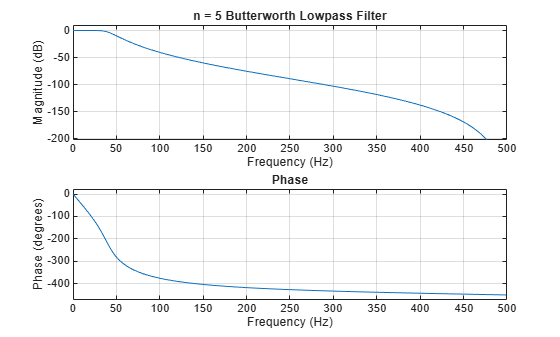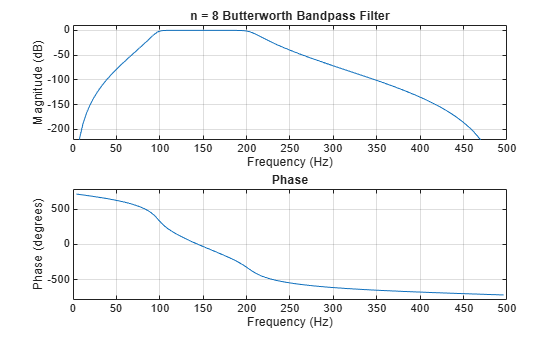Documentation

This is machine translation

Mouseover text to see original. Click the button below to return to the English version of the page.

buttord

Butterworth filter order and cutoff frequency

Syntax

``[n,Wn] = buttord(Wp,Ws,Rp,Rs)``
``[n,Wn] = buttord(Wp,Ws,Rp,Rs,'s')``

Description

example

````[n,Wn] = buttord(Wp,Ws,Rp,Rs)` returns the lowest order, `n`, of the digital Butterworth filter with no more than `Rp` dB of passband ripple and at least `Rs` dB of attenuation in the stopband. `Wp` and `Ws` are respectively the passband and stopband edge frequencies of the filter, normalized from 0 to 1, where 1 corresponds to π rad/sample. The scalar (or vector) of corresponding cutoff frequencies, `Wn`, is also returned. To design a Butterworth filter, use the output arguments `n` and `Wn` as inputs to `butter`.```
````[n,Wn] = buttord(Wp,Ws,Rp,Rs,'s')` finds the minimum order `n` and cutoff frequencies `Wn` for an analog Butterworth filter. Specify the frequencies `Wp` and `Ws` in radians per second. The passband or the stopband can be infinite.```

Examples

collapse all

For data sampled at 1000 Hz, design a lowpass filter with no more than 3 dB of ripple in a passband from 0 to 40 Hz, and at least 60 dB of attenuation in the stopband. Find the filter order and cutoff frequency.

```Wp = 40/500; Ws = 150/500; [n,Wn] = buttord(Wp,Ws,3,60)```
```n = 5 ```
```Wn = 0.0810 ```

Specify the filter in terms of second-order sections and plot the frequency response.

```[z,p,k] = butter(n,Wn); sos = zp2sos(z,p,k); freqz(sos,512,1000) title(sprintf('n = %d Butterworth Lowpass Filter',n))```Design a bandpass filter with a passband from 100 to 200 Hz with at most 3 dB of passband ripple and at least 40 dB attenuation in the stopbands. Specify a sample rate of 1 kHz. Set the stopband width to 50 Hz on both sides of the passband. Find the filter order and cutoff frequencies.

```Wp = [100 200]/500; Ws = [50 250]/500; Rp = 3; Rs = 40; [n,Wn] = buttord(Wp,Ws,Rp,Rs)```
```n = 8 ```
```Wn = 1×2 0.1951 0.4080 ```

Specify the filter in terms of second-order sections and plot the frequency response.

```[z,p,k] = butter(n,Wn); sos = zp2sos(z,p,k); freqz(sos,128,1000) title(sprintf('n = %d Butterworth Bandpass Filter',n))```Input Arguments

collapse all

Passband corner (cutoff) frequency, specified as a scalar or a two-element vector with values between 0 and 1, with 1 corresponding to the normalized Nyquist frequency, π rad/sample.

• If `Wp` and `Ws` are both scalars and `Wp` < `Ws`, then `buttord` returns the order and cutoff frequency of a lowpass filter. The stopband of the filter ranges from `Ws` to 1 and the passband ranges from 0 to `Wp`.

• If `Wp` and `Ws` are both scalars and `Wp` > `Ws`, then `buttord` returns the order and cutoff frequency of a highpass filter. The stopband of the filter ranges from 0 to `Ws` and the passband ranges from `Wp` to 1.

• If `Wp` and `Ws` are both vectors and the interval specified by `Ws` contains the one specified by `Wp` (`Ws(1)` < `Wp(1)` < `Wp(2)` < `Ws(2)`), then `buttord` returns the order and cutoff frequencies of a bandpass filter. The stopband of the filter ranges from 0 to `Ws(1)` and from `Ws(2)` to 1. The passband ranges from `Wp(1)` to `Wp(2)`.

• If `Wp` and `Ws` are both vectors and the interval specified by `Wp` contains the one specified by `Ws` (`Wp(1)` < `Ws(1)` < `Ws(2)` < `Wp(2)`), then `buttord` returns the order and cutoff frequencies of a bandstop filter. The stopband of the filter ranges from `Ws(1)` to `Ws(2)`. The passband ranges from 0 to `Wp(1)` and from `Wp(2)` to 1.

Data Types: `single` | `double`

Note

If your filter specifications call for a bandpass or bandstop filter with unequal ripple in each of the passbands or stopbands, design separate lowpass and highpass filters and cascade the two filters together.

Stopband corner frequency, specified as a scalar or a two-element vector with values between 0 and 1, with 1 corresponding to the normalized Nyquist frequency, π rad/sample.

Data Types: `single` | `double`

Passband ripple, specified as a scalar expressed in dB.

Data Types: `single` | `double`

Stopband attenuation, specified as a scalar expressed in dB.

Data Types: `single` | `double`

Output Arguments

collapse all

Lowest filter order, returned as an integer scalar.

Cutoff frequencies, returned as a scalar or vector.

Algorithms

`buttord`’s order prediction formula operates in the analog domain for both analog and digital cases. For the digital case, it converts the frequency parameters to the s-domain before estimating the order and natural frequency. The function then converts back to the z-domain.

`buttord` initially develops a lowpass filter prototype by transforming the passband frequencies of the desired filter to 1 rad/second (for lowpass and highpass filters) and to –1 and 1 rad/second (for bandpass and bandstop filters). It then computes the minimum order required for a lowpass filter to meet the stopband specification.

 Rabiner, Lawrence R., and Bernard Gold. Theory and Application of Digital Signal Processing. Englewood Cliffs, NJ: Prentice-Hall, 1975.